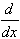#Interactive Real Analysis

Next | Previous | Glossary | Map

## 7.1. Riemann Integral

### Examples 7.1.21(b):

Find the value of the following integrals:
1.x5 - 4 x2 dx on the interval [0, 2].
2.1/x2 + cos(x) dx on the interval [1, 4].
3.(1 + x2)-1 dx on the interval [-1, 1].
This time we will use the Integral Evaluation Shortcut, or First Fundamental Theorem of Calculus, which requires us to find the antiderivative for each of the functions.

1. Here is a numerical approximation ofx5 - 4 x2 dx on the interval [0, 2].

To compute the exact value, we let
P(x) = 1/6 x6 - 4/3 x3 + C
Then we clearly have:
P'(x) = x5 - 4 x2
so that P is an antiderivate of the integrand. Therefore:x5 - 4 x2 dx = P(b) - P(a)
With our choices of a and b we can evaluate the integral to
P(2) - P(0) = 1/6 26 - 4/3 23 = 1/6 64 - 4/3 * 8 = 0

2. Here is a numerical approximation of1/x2 + cos(x) dx on the interval [1, 4].

To compute the exact value, first note that 1/x2 + cos(x) is continuous over the interval [-1, 4] so that the function is Riemann integrable. If we define P(x) = -1/x + sin(x) + C then P'(x) = 1/x2 + cos(x) so that again we found an antiderivative of the original integrand. Using the Integral Evaluation Shortcut over [-1, 4] we can compute the value of the integral to
P(4) - P(1) = -1/4 + sin(4) - (-1/1 + sin(1)) = -.8482734801

3. Here is a numerical approximation of(1 + x2)-1 dx on the interval [-1, 1].

The integrand is continuous everywhere, so that it is Riemann integrable over any closed bounded interval. To find an antiderivative of (1 + x2)-1 is less obvious than in the previous cases until we remember thatarctan(x) = (1 + x2)-1
But then the integral evaluates to
arctan(1) - arctan(-1) = 2/4 =/2 = 1.570796327
Next | Previous | Glossary | Map